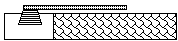## FINAL PROJECT

Modeling Patterns in Experimental Data

Many of the most important algebraic forms arise as models of relationships among scientific variables. Of course, by the time those relations make their way to the mathematics classroom, they are usually well-defined and relatively simple expressions. The mathematical modeling experience in real life is a more complex process--identifying variables, running experiments that yield data, and finding algebraic expressions that summarize patterns in the data. The following examples illustrate ways that such a process can occur.

In preparation for question 2 on the MATH 110 final examination, join with your group partners to select and run one of the following experiments that lead eventually to algebraic models of quantitative relationships.

1. Begin this experiment by tossing about 10 coins onto a flat surface. Count the number of coins that land with heads down and add that number of coins to the test supply. Note the number of coins added and the new total of coins in your test supply. Repeat the toss-count-add process to a total of at least ten trials.

(a) Record the data in a table of data like this:

```Toss number          0     1       2       3       4       5       6       7
Total Number of      10                    ...
Coins

```

(b) Plot the (toss number, total number of coins) data.

(c) Describe and try to explain the pattern observed.

2. One of the most important scientific principles is illustrated in the typical playground teeter-totter or see-saw. It also comes into play when a diver bounces on the end of a diving board.(a) What sort of relation would you expect between the length of a diving board and the weight that it could support before breaking? Sketch a graph of your conjecture about the relation between length and breaking weight..

(b) Using some pieces of fettuccini, a cup hung at the end of the fettuccini diving board, and some pennies, collect data relating length to breaking weight:

```Length of "board" cm          24     20          16       12     8          4
Breaking weight pennies

```

(c) Find an equation that models well the relation between length and breaking weight.